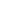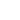AI

# 机器学习开放课程：五、Bagging与随机森林

【编者按】机器学习开放课程第五课，Mail.Ru数据科学家Yury Kashnitsky和You Scan数据科学家Vitaliy Radchenko深入浅出地介绍了集成、Bagging、随机森林、特征重要性。#### 概览

1. 集成
2. Bootstraping
3. Bagging
4. 袋外误差
5. 随机森林
6. 特征重要性
7. 相关资源

#### 1. 集成

• N为陪审员总数；
• m是构成多数的最小值，即m = (N+1)/2；
• p为评审员做出正确决策的概率；
• μ是整个评审团做出正确决策的概率。#### 2. Bootstraping

Leo Breiman于1994年提出的Bagging（又称Bootstrap aggregation，引导聚集）是最基本的集成技术之一。Bagging基于统计学中的bootstraping（自助法），该方法使得评估许多复杂模型的统计数据更可行。

bootstrap方法的流程如下：假设有尺寸为N的样本X。我们可以从该样本中有放回地随机均匀抽取N个样本，以创建一个新样本。换句话说，我们从尺寸为N的原样本中随机选择一个元素，并重复此过程N次。选中所有元素的可能性是一样的，因此每个元素被抽中的概率均为1/N``````import pandas as pd
from matplotlib import pyplot as plt
plt.style.use('ggplot')
plt.rcParams['figure.figsize'] = 10, 6
import seaborn as sns
%matplotlib inline
telecom_data = pd.read_csv('../../data/telecom_churn.csv')
fig = sns.kdeplot(telecom_data[telecom_data['Churn'] == False]['Customer service calls'],
label = 'Loyal')
fig = sns.kdeplot(telecom_data[telecom_data['Churn'] == True]['Customer service calls'],
label = 'Churn')
fig.set(xlabel='Number of calls', ylabel='Density')
plt.show()
````````````import numpy as np
def get_bootstrap_samples(data, n_samples):
"""使用bootstrap方法生成bootstrap样本。"""
indices = np.random.randint(0, len(data), (n_samples, len(data)))
samples = data[indices]
return samples
def stat_intervals(stat, alpha):
"""生成区间估计。"""
boundaries = np.percentile(stat, [100 * alpha / 2., 100 * (1 - alpha / 2.)])
return boundaries
``````

``````loyal_calls = telecom_data[telecom_data['Churn']
== False]['Customer service calls'].values
churn_calls= telecom_data[telecom_data['Churn']
== True]['Customer service calls'].values
``````

``````np.random.seed(0)
``````

``````loyal_mean_scores = [np.mean(sample)
for sample in get_bootstrap_samples(loyal_calls, 1000)]
churn_mean_scores = [np.mean(sample)
for sample in get_bootstrap_samples(churn_calls, 1000)]
``````

``````print("忠实客户呼叫客服数: 均值区间",
stat_intervals(loyal_mean_scores, 0.05))
print("离网客户呼叫客服数：均值区间",
stat_intervals(churn_mean_scores, 0.05))
``````

``````忠实客户呼叫客服数: 均值区间 [1.4077193 1.49473684]

``````

#### 3. Baggingbagging通过在不同数据集上训练模型降低分类器的方差。换句话说，bagging可以预防过拟合。bagging的有效性来自不同训练数据集上单独模型的不同，它们的误差在投票过程中相互抵消。此外，某些bootstrap训练样本很可能略去离散值。#### 4. 袋外误差#### 5. 随机森林

Leo Breiman不仅将bootstrap应用于统计，同时也将其应用于机器学习。他和Adel Cutler扩展并改进了Tin Kam Ho提出的的随机森林算法。他们组合使用CART、bagging、随机子空间方法构建无关树。

1. 设样本数等于n，特征维度数等于d

2. 选择集成中单个模型的数目M

3. 对于每个模型m，选择特征数dm < d。所有模型使用相同的dm值。

4. 对每个模型m，通过在整个d特征集合上随机选择dm个特征创建一个训练集。

5. 训练每个模型。

6. 通过组合M中的所有模型的结果，应用所得集成模型于新输入。可以使用大多数投票（majority voting）或后验概率加总（aggregation of the posterior probabilities）。

5.1 算法

• 生成bootstrap样本Xk

• 在样本Xk上创建一棵决策树bk

• 根据给定的标准选择最佳的特征维度。根据该特征分割样本以创建树的新层次。重复这一流程，直到竭尽样本。

• 创建树，直到任何叶节点包含不超过nmin个实例，或者达到特定深度。

• 对每个分割，我们首先从d个原始特征中随机选择m个特征，接着只在该子集上搜索最佳分割。5.2 与决策树和bagging的比较

``````import warnings
import numpy as np
warnings.filterwarnings('ignore')

%matplotlib inline
from matplotlib import pyplot as plt
plt.style.use('ggplot')
plt.rcParams['figure.figsize'] = 10, 6
import seaborn as sns
from sklearn.ensemble import RandomForestRegressor, RandomForestClassifier
from sklearn.ensemble import BaggingClassifier, BaggingRegressor
from sklearn.tree import DecisionTreeRegressor, DecisionTreeClassifier
from sklearn.datasets import make_circles
from sklearn.model_selection import train_test_split

n_train = 150
n_test = 1000
noise = 0.1
``````

``````def f(x):
x = x.ravel()
return np.exp(-x ** 2) + 1.5 * np.exp(-(x - 2) ** 2)

def generate(n_samples, noise):
X = np.random.rand(n_samples) * 10 - 5
X = np.sort(X).ravel()
y = np.exp(-X ** 2) + 1.5 * np.exp(-(X - 2) ** 2)\
+ np.random.normal(0.0, noise, n_samples)
X = X.reshape((n_samples, 1))

return X, y

X_train, y_train = generate(n_samples=n_train, noise=noise)
X_test, y_test = generate(n_samples=n_test, noise=noise)
``````

``````dtree = DecisionTreeRegressor().fit(X_train, y_train)
d_predict = dtree.predict(X_test)

plt.figure(figsize=(10, 6))
plt.plot(X_test, f(X_test), "b")
plt.scatter(X_train, y_train, c="b", s=20)
plt.plot(X_test, d_predict, "g", lw=2)
plt.xlim([-5, 5])
plt.title("Decision tree, MSE = %.2f"
% np.sum((y_test - d_predict) ** 2))
``````

``````bdt = BaggingRegressor(DecisionTreeRegressor()).fit(X_train, y_train)
bdt_predict = bdt.predict(X_test)

plt.figure(figsize=(10, 6))
plt.plot(X_test, f(X_test), "b")
plt.scatter(X_train, y_train, c="b", s=20)
plt.plot(X_test, bdt_predict, "y", lw=2)
plt.xlim([-5, 5])
plt.title("Bagging for decision trees, MSE = %.2f" % np.sum((y_test - bdt_predict) ** 2));
``````

``````rf = RandomForestRegressor(n_estimators=10).fit(X_train, y_train)
rf_predict = rf.predict(X_test)

plt.figure(figsize=(10, 6))
plt.plot(X_test, f(X_test), "b")
plt.scatter(X_train, y_train, c="b", s=20)
plt.plot(X_test, rf_predict, "r", lw=2)
plt.xlim([-5, 5])
plt.title("Random forest, MSE = %.2f" % np.sum((y_test - rf_predict) ** 2));
````````````np.random.seed(42)
X, y = make_circles(n_samples=500, factor=0.1, noise=0.35, random_state=42)
X_train_circles, X_test_circles, y_train_circles, y_test_circles = train_test_split(X, y, test_size=0.2)

dtree = DecisionTreeClassifier(random_state=42)
dtree.fit(X_train_circles, y_train_circles)

x_range = np.linspace(X.min(), X.max(), 100)
xx1, xx2 = np.meshgrid(x_range, x_range)
y_hat = dtree.predict(np.c_[xx1.ravel(), xx2.ravel()])
y_hat = y_hat.reshape(xx1.shape)
plt.contourf(xx1, xx2, y_hat, alpha=0.2)
plt.scatter(X[:,0], X[:,1], c=y, cmap='autumn')
plt.title("Decision tree")
plt.show()

b_dtree = BaggingClassifier(DecisionTreeClassifier(),n_estimators=300, random_state=42)
b_dtree.fit(X_train_circles, y_train_circles)

x_range = np.linspace(X.min(), X.max(), 100)
xx1, xx2 = np.meshgrid(x_range, x_range)
y_hat = b_dtree.predict(np.c_[xx1.ravel(), xx2.ravel()])
y_hat = y_hat.reshape(xx1.shape)
plt.contourf(xx1, xx2, y_hat, alpha=0.2)
plt.scatter(X[:,0], X[:,1], c=y, cmap='autumn')
plt.title("Bagging (decision trees)")
plt.show()

rf = RandomForestClassifier(n_estimators=300, random_state=42)
rf.fit(X_train_circles, y_train_circles)

x_range = np.linspace(X.min(), X.max(), 100)
xx1, xx2 = np.meshgrid(x_range, x_range)
y_hat = rf.predict(np.c_[xx1.ravel(), xx2.ravel()])
y_hat = y_hat.reshape(xx1.shape)
plt.contourf(xx1, xx2, y_hat, alpha=0.2)
plt.scatter(X[:,0], X[:,1], c=y, cmap='autumn')
plt.title("Random forest")
plt.show()
``````5.3 参数

`scikit-learn`库提供了`BaggingRegressor``BaggingClassifier`

• `n_estimators`是森林中树的数量；
• `criterion`是衡量分割质量的函数；
• `max_features`是查找最佳分割时考虑的特征数；
• `min_samples_leaf`是叶节点的最小样本数；
• `max_depth`是树的最大深度。

``````import pandas as pd
from sklearn.model_selection import cross_val_score, StratifiedKFold, GridSearchCV
from sklearn.metrics import accuracy_score

df = pd.read_csv("../../data/telecom_churn.csv")
``````

``````cols = []
for i in df.columns:
if (df[i].dtype == "float64") or (df[i].dtype == 'int64'):
cols.append(i)
``````

``````X, y = df[cols].copy(), np.asarray(df["Churn"],dtype='int8')
``````

``````skf = StratifiedKFold(n_splits=5, shuffle=True, random_state=42)
``````

``````rfc = RandomForestClassifier(random_state=42, n_jobs=-1, oob_score=True)
``````

``````results = cross_val_score(rfc, X, y, cv=skf)
``````

``````print("交叉验证精确度评分： {:.2f}%".format(results.mean()*100))
``````

``````交叉验证精确度评分：91.48%
``````

``````skf = StratifiedKFold(n_splits=5, shuffle=True, random_state=42)
``````

``````train_acc = []
test_acc = []
temp_train_acc = []
temp_test_acc = []
``````

``````trees_grid = [5, 10, 15, 20, 30, 50, 75, 100]
``````

``````for ntrees in trees_grid:
rfc = RandomForestClassifier(n_estimators=ntrees, random_state=42, n_jobs=-1, oob_score=True)
temp_train_acc = []
temp_test_acc = []
for train_index, test_index in skf.split(X, y):
X_train, X_test = X.iloc[train_index], X.iloc[test_index]
y_train, y_test = y[train_index], y[test_index]
rfc.fit(X_train, y_train)
temp_train_acc.append(rfc.score(X_train, y_train))
temp_test_acc.append(rfc.score(X_test, y_test))
train_acc.append(temp_train_acc)
test_acc.append(temp_test_acc)
``````

``````train_acc, test_acc = np.asarray(train_acc), np.asarray(test_acc)
print("交叉验证最佳精确度为 {:.2f}% 在 {} 树时达到".format(max(test_acc.mean(axis=1))*100,
trees_grid[np.argmax(test_acc.mean(axis=1))]))
``````

``````交叉验证最佳精确度为 92.44% 在 50 树时达到
``````

``````plt.style.use('ggplot')

fig, ax = plt.subplots(figsize=(8, 4))
ax.plot(trees_grid, train_acc.mean(axis=1), alpha=0.5, color='blue', label='train')
ax.plot(trees_grid, test_acc.mean(axis=1), alpha=0.5, color='red', label='cv')
ax.fill_between(trees_grid, test_acc.mean(axis=1) - test_acc.std(axis=1), test_acc.mean(axis=1) + test_acc.std(axis=1), color='#888888', alpha=0.4)
ax.fill_between(trees_grid, test_acc.mean(axis=1) - 2*test_acc.std(axis=1), test_acc.mean(axis=1) + 2*test_acc.std(axis=1), color='#888888', alpha=0.2)
ax.legend(loc='best')
ax.set_ylim([0.88,1.02])
ax.set_ylabel("Accuracy")
ax.set_xlabel("N_estimators");
````````````train_acc = []
test_acc = []
temp_train_acc = []
temp_test_acc = []
max_depth_grid = [3, 5, 7, 9, 11, 13, 15, 17, 20, 22, 24]

for max_depth in max_depth_grid:
rfc = RandomForestClassifier(n_estimators=100, random_state=42, n_jobs=-1, oob_score=True, max_depth=max_depth)
temp_train_acc = []
temp_test_acc = []
for train_index, test_index in skf.split(X, y):
X_train, X_test = X.iloc[train_index], X.iloc[test_index]
y_train, y_test = y[train_index], y[test_index]
rfc.fit(X_train, y_train)
temp_train_acc.append(rfc.score(X_train, y_train))
temp_test_acc.append(rfc.score(X_test, y_test))
train_acc.append(temp_train_acc)
test_acc.append(temp_test_acc)

train_acc, test_acc = np.asarray(train_acc), np.asarray(test_acc)
print("交叉验证最佳精确度为 {:.2f}% 当 max_depth 为 {} 时达到".format(max(test_acc.mean(axis=1))*100,
max_depth_grid[np.argmax(test_acc.mean(axis=1))]))
``````

``````交叉验证最佳精确度为 92.68% 当 max_depth 为 17 时达到
``````
``````fig, ax = plt.subplots(figsize=(8, 4))
ax.plot(max_depth_grid, train_acc.mean(axis=1), alpha=0.5, color='blue', label='train')
ax.plot(max_depth_grid, test_acc.mean(axis=1), alpha=0.5, color='red', label='cv')
ax.fill_between(max_depth_grid, test_acc.mean(axis=1) - test_acc.std(axis=1), test_acc.mean(axis=1) + test_acc.std(axis=1), color='#888888', alpha=0.4)
ax.fill_between(max_depth_grid, test_acc.mean(axis=1) - 2*test_acc.std(axis=1), test_acc.mean(axis=1) + 2*test_acc.std(axis=1), color='#888888', alpha=0.2)
ax.legend(loc='best')
ax.set_ylim([0.88,1.02])
ax.set_ylabel("Accuracy")
ax.set_xlabel("Max_depth");
```````max_depth`在我们的模型中起到了正则化的作用，模型不像之前过拟合得那么严重了。模型精确度略有提升。

``````train_acc = []
test_acc = []
temp_train_acc = []
temp_test_acc = []
min_samples_leaf_grid = [1, 3, 5, 7, 9, 11, 13, 15, 17, 20, 22, 24]

for min_samples_leaf in min_samples_leaf_grid:
rfc = RandomForestClassifier(n_estimators=100, random_state=42, n_jobs=-1,
oob_score=True, min_samples_leaf=min_samples_leaf)
temp_train_acc = []
temp_test_acc = []
for train_index, test_index in skf.split(X, y):
X_train, X_test = X.iloc[train_index], X.iloc[test_index]
y_train, y_test = y[train_index], y[test_index]
rfc.fit(X_train, y_train)
temp_train_acc.append(rfc.score(X_train, y_train))
temp_test_acc.append(rfc.score(X_test, y_test))
train_acc.append(temp_train_acc)
test_acc.append(temp_test_acc)

train_acc, test_acc = np.asarray(train_acc), np.asarray(test_acc)
print("交叉验证最佳精确度为 {:.2f}% 当 min_samples_leaf 为 {} 时达到".format(max(test_acc.mean(axis=1))*100,
min_samples_leaf_grid[np.argmax(test_acc.mean(axis=1))]))
``````

``````交叉验证最佳精确度为 92.41% 当 min_samples_leaf 为 3 时达到
``````
``````fig, ax = plt.subplots(figsize=(8, 4))
ax.plot(min_samples_leaf_grid, train_acc.mean(axis=1), alpha=0.5, color='blue', label='train')
ax.plot(min_samples_leaf_grid, test_acc.mean(axis=1), alpha=0.5, color='red', label='cv')
ax.fill_between(min_samples_leaf_grid, test_acc.mean(axis=1) - test_acc.std(axis=1), test_acc.mean(axis=1) + test_acc.std(axis=1), color='#888888', alpha=0.4)
ax.fill_between(min_samples_leaf_grid, test_acc.mean(axis=1) - 2*test_acc.std(axis=1), test_acc.mean(axis=1) + 2*test_acc.std(axis=1), color='#888888', alpha=0.2)
ax.legend(loc='best')
ax.set_ylim([0.88,1.02])
ax.set_ylabel("Accuracy")
ax.set_xlabel("Min_samples_leaf");
````````````train_acc = []
test_acc = []
temp_train_acc = []
temp_test_acc = []
max_features_grid = [2, 4, 6, 8, 10, 12, 14, 16]

for max_features in max_features_grid:
rfc = RandomForestClassifier(n_estimators=100, random_state=42, n_jobs=-1,
oob_score=True, max_features=max_features)
temp_train_acc = []
temp_test_acc = []
for train_index, test_index in skf.split(X, y):
X_train, X_test = X.iloc[train_index], X.iloc[test_index]
y_train, y_test = y[train_index], y[test_index]
rfc.fit(X_train, y_train)
temp_train_acc.append(rfc.score(X_train, y_train))
temp_test_acc.append(rfc.score(X_test, y_test))
train_acc.append(temp_train_acc)
test_acc.append(temp_test_acc)

train_acc, test_acc = np.asarray(train_acc), np.asarray(test_acc)
print("交叉验证最佳精确度为 {:.2f}% 当 max_features 为 {} 时达到".format(max(test_acc.mean(axis=1))*100,
max_features_grid[np.argmax(test_acc.mean(axis=1))]))
``````

``````交叉验证最佳精确度为 92.59% 当 max_features 为 10 时达到
``````
``````fig, ax = plt.subplots(figsize=(8, 4))
ax.plot(max_features_grid, train_acc.mean(axis=1), alpha=0.5, color='blue', label='train')
ax.plot(max_features_grid, test_acc.mean(axis=1), alpha=0.5, color='red', label='cv')
ax.fill_between(max_features_grid, test_acc.mean(axis=1) - test_acc.std(axis=1), test_acc.mean(axis=1) + test_acc.std(axis=1), color='#888888', alpha=0.4)
ax.fill_between(max_features_grid, test_acc.mean(axis=1) - 2*test_acc.std(axis=1), test_acc.mean(axis=1) + 2*test_acc.std(axis=1), color='#888888', alpha=0.2)
ax.legend(loc='best')
ax.set_ylim([0.88,1.02])
ax.set_ylabel("Accuracy")
ax.set_xlabel("Max_features");
````````````parameters = {'max_features': [4, 7, 10, 13], 'min_samples_leaf': [1, 3, 5, 7], 'max_depth': [5,10,15,20]}
rfc = RandomForestClassifier(n_estimators=100, random_state=42,
n_jobs=-1, oob_score=True)
gcv = GridSearchCV(rfc, parameters, n_jobs=-1, cv=skf, verbose=1)
gcv.fit(X, y)

gcv.best_estimator_, gcv.best_score_
``````

``````(RandomForestClassifier(bootstrap=True, class_weight=None, criterion='gini',
max_depth=10, max_features=10, max_leaf_nodes=None,
min_impurity_decrease=0.0, min_impurity_split=None,
min_samples_leaf=1, min_samples_split=2,
min_weight_fraction_leaf=0.0, n_estimators=100, n_jobs=-1,
oob_score=True, random_state=42, verbose=0, warm_start=False),
0.9270927092709271)
``````

5.4 方差和去相关• p(x)为任何两棵树之间的样本相关性；• Θ1(Z)Θ2(Z)为样本Z上随机选择的元素上随机选择的一对树；

• T(x, Θi(Z))为第i个树分类器在输入向量x上的输出；

• σ2(x)为任何随机选择的树上的样本方差：5.5 偏差5.6 极端随机树

`scikit-learn`库实现了[ ExtraTreesClassifier]和ExtraTreesRegressor

5.7 随机森林和k近邻的相似性1. 基于随机森林或梯度提升技术在样本上训练较小数目的决策树的复合模型

2. 将类别特征T1(x),…,Tn(x)加入样本5.8 转换数据集为高维表示

5.9 随机森林的优势和劣势

• 高预测精确度；在大多数问题上表现优于线性算法；精确度与boosting相当；

• 多亏了随机取样，对离散值的鲁棒性较好；

• 随机子空间选取导致对特征缩放及其他单调转换不敏感；

• 不需要精细的参数调整，开箱即用。取决于问题设定和数据，调整参数可能取得0.5%到3%的精确度提升；

• 在具有大量特征和分类的数据集上很高效；

• 既可处理连续值，也可处理离散值；

• 罕见过拟合。在实践中，增加树的数量几乎总是能提升总体表现。不过，当达到特定数量后，学习曲线非常接近渐近线；

• 有成熟方法用于估计特征重要性；

• 能够很好地处理数据缺失，即使当很大一部分数据缺失时，仍能保持较好的精确度；

• 支持整个数据集及单棵树样本上的加权分类；

• 决策树底层使用的实例亲近性计算可以在后续用于聚类、检测离散值、感兴趣数据表示；

• 以上功能和性质可以扩展到未标注数据，以支持无监督聚类，数据可视化和离散值检测；

• 易于并行化，伸缩性强。

• 相比单棵决策树，随机森林的输出更难解释。

• 特征重要性估计没有形式化的p值。

• 在稀疏数据情形（比如，文本输入、词袋）下，表现不如线性模型好。

• 和线性回归不同，随机森林无法外推。不过，这也可以看成优势，因为离散值不会在随机森林中导致极端值。

• 在某些问题上容易过拟合，特别是处理高噪声数据。

• 处理数量级不同的类别数据时，随机森林偏重数量级较高的变量，因为这能提高更多精确度；

• 如果数据集包含对预测分类重要度相似的相关特征分组，那么随机森林将偏重较小的分组；

• 所得模型较大，需要大量RAM。

#### 6. 特征重要性• 未经正则化：• 使用标准差正则化后：``````import warnings
warnings.filterwarnings('ignore')

%matplotlib inline
from matplotlib import pyplot as plt
import seaborn as sns

from matplotlib import rc
font = {'family': 'Verdana',
'weight': 'normal'}
rc('font', **font)

import pandas as pd
import numpy as np
from sklearn.ensemble.forest import RandomForestRegressor

hostel_data = pd.read_csv("../../data/hostel_factors.csv")
features = {"f1":u"Staff",
"f2":u"Hostel booking",
"f3":u"Check-in and check-out",
"f4":u"Room condition",
"f5":u"Shared kitchen condition",
"f6":u"Shared space condition",
"f7":u"Extra services",
"f8":u"General conditions & conveniences",
"f9":u"Value for money",
"f10":u"Customer Co-creation"}

forest = RandomForestRegressor(n_estimators=1000, max_features=10,
random_state=0)

forest.fit(hostel_data.drop(['hostel', 'rating'], axis=1),
hostel_data['rating'])
importances = forest.feature_importances_

indices = np.argsort(importances)[::-1]

num_to_plot = 10
feature_indices = [ind+1 for ind in indices[:num_to_plot]]

plt.figure(figsize=(15,5))
plt.title(u"Feature Importance")
bars = plt.bar(range(num_to_plot),
importances[indices[:num_to_plot]],
color=([str(i/float(num_to_plot+1))
for i in range(num_to_plot)]),
align="center")
ticks = plt.xticks(range(num_to_plot),
feature_indices)
plt.xlim([-1, num_to_plot])
plt.legend(bars, [u''.join(features["f"+str(i)])
for i in feature_indices]);
``````#### 7. 相关资源

• Jerome H. Friedman、Robert Tibshirani、Trevor Hastie著Elements of Statistical Learning第15章。

• `scikit-learn`库的官方文档提供了更多关于随机森林和其他集成算法的应用示例。

• 如果想要深入了解随机森林的方差和去相关，请参阅原始论文

### 评论游客2018-01-07

2017-10-17

2017-12-25

2017-07-24

### 告别曲线拟合：因果推断和do-Calculus简介发布需求联系客服